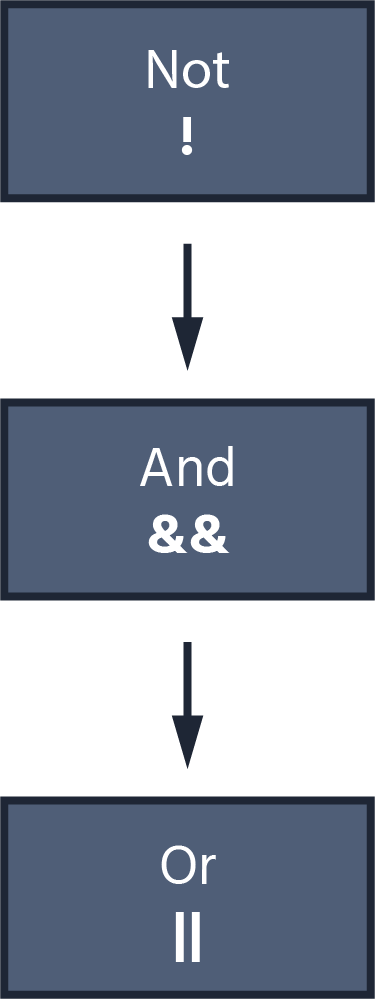Course Content

Introduction to JavaScript

##Logical Operations

Now, let's explore logical operations. Logical operations are used to manipulate and make decisions based on boolean values.

# Logical Operators

There are three logical operators in JavaScript:

• AND (`&&`);
• OR (`||`);
• NOT (`!`).

### AND (&&)

The AND (`&&`) logical operator returns `true` only when both values it operates on are `true`. The result is `false` if any value is `false`.The AND (`&&`) operator requires all values to be `true` to return `true`.

### OR (||)

The OR (`||`) logical operator returns `true` if at least one of the values is `true`. It returns `false` only when both values are `false`.The OR (`||`) operator returns `false` only when both values are `false`.

### NOT (!)

The NOT (`!`) operator inverts the boolean value. It turns `true` into `false` and `false` into `true`.# Using Logical Operators

Logical operators are often used to combine conditions. For example, to check if two values are greater than `4` or greater than `1`, you can use the AND (`&&`) operator.# Priority

Logical operations have a lower priority than comparison operations, which, in turn, have a lower priority than mathematical operations.Note

Similar to other operations, you can use parentheses `()` to adjust the priority of execution.

1. What will be the output?
2. What will be the output?
3. What will be the output?